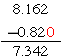#You have learned to add, subtract, multiply, and divide decimal numbers. Now, we will extend that knowledge to include negative and positive decimal numbers and multi-step problems that involve operations with decimals.

It’s Simple!

Addition and subtraction of decimal numbers involves simply lining up the decimals all the way through the problem.

For example, with the numbers 8.162 and 0.82:

8.162        8.162
– 0.820     + 0.820
7.342        8.982

Now, switch the signs up a bit and do the same thing using your integer addition and subtraction rules!

8.162 + (-0.820)

Would be written as:8.162 – (-0.820)

Would be written as:## Multiply Decimal Numbers

Remember that the answer to a multiplication problem is called the product of the factors. To multiply numbers with decimals do not worry about placement, just multiply the numbers, then place the decimal point correctly in the product by counting the decimal places in each of the factors and counting that many total place from the right for the product.

### Examples

2.3 * 5.06 = 11.638  (1+ 2 places = 3 places from the right)

0.0004 * 3.42 = 0.001368  (4 + 2 places = 6 places from the right)

At this point, we are simply going to learn to solve these problems by following the rules we learned for multiplication of negative numbers. These rules state that when both factors have the same sign, the product is positive, and when the factors have different signs, the product is negative.

–  2.3 * 5.06 = 11.638  (1+ 2 places = 3 places from the right – product is negative)

–  0.0004 * (- 3.42) = 0.001368  (4 + 2 places = 6 places from the right – product is positive)

## Division

Let’s review the process for dividing by a decimal number.

1. When you are dividing by a decimal number, count how many digits the decimal point must move to the right to become an integer. (Remember, you cannot divide by a number with a decimal). If the number is an integer, just leave the decimal in the dividend alone.
2. Move the decimal point in the dividend (in the box) the same direction and the same number of digits.
3. Remember your division rule for signed numbers is the same as the multiplication rule.

### Example(Different signs will equal a negative number)

Now that we have reviewed our decimal operations rules and learned how to include our negative and positive number operation rules in solving these problems, lets take a look at some word problems.

• The number of gallons of water that leak from Steve’s pool can be represented by -0.3. Steve uses jars that hold 0.05 gallon. How many jars will it take to hold all of the water leaking from the pool?
• In this problem, you will need to divide the total amount of water leaking from the pool by the amount of water held by each jar. This will tell you how many jars he will need to catch the leak.

How did you do? Did you remember to move the decimal of both numbers as you completed your long division? Did you remember that the answer was positive because both the divisor and the dividend were negative?

### Example

• Rebeccas bank statement shows a deduction of -\$1.50 taken out of her bank account every week. How many weeks will it take before the total deduction is -\$12? Work it out and see if you selected the right operation and sign.### One More Example!

Jordan ran 3.2 miles downhill every day after walking up to the top of the hill. He did this for 21 days last month. How many miles did he jog last month? Check your answer!

(source)